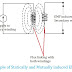In Simple words, the EMF or Electromotive force is generated when there is a change in the magnetic field passing through a conductor(such as a wire loop) or the changes in the conductor associated with a constant magnetic field.

This change in magnetic field can occur due to different reasons such as Magnetic Field Variation, Magnetic Field Movement, Changing the position and Area of the Conductor, and Time-Varying Magnetic Field.

### Magnetic Field Variation

When a magnetic field passing through a conductor changes, an EMF is induced in the conductor. This change can occur by moving a magnet near the conductor, altering the strength of the magnetic field, or changing the orientation of the magnetic field lines with respect to the conductor.

### Conductor Movement

Similarly, if a conductor is moved across a stationary magnetic field, an EMF is induced. This is known as motion-induced EMF or generator effect. It is the principle behind electric generators and alternators that convert mechanical energy into electrical energy.

### Changing the Area of the Conductor

If the area of a wire loop or a conductor is varied while a magnetic field remains constant, an EMF is induced. This effect can be seen in some devices such as inductive sensors.

### Time-Varying Magnetic Field

A time-varying magnetic field that occurs due to an alternating current (AC) also can induce an EMF in a conductor. This is the principle behind the operation of transformers and the generation of AC electricity.

According to Faraday's law, the magnitude of the induced EMF is directly proportional to the rate of change of magnetic field or flux and the number of turns in the conductor. This relationship is expressed by the equation:

EMF = - dΦ/dt

Where EMF represents the induced electromotive force, dΦ/dt represents the rate of change of magnetic flux, and the negative sign indicates the direction of the induced current opposes the change in magnetic field.

## Types of induced EMF

There are two types of Induced EMF,

1. Statically Induced EMF
2. Dynamically Induced EMF

Statically Induced EMF is two types,

1. Self-induced EMF
2. Mutually induced EMF

### Statically Induced EMF:

If a stationary conductor(conductor does not change its position) is placed in a changing magnetic field as a result of the flux linkage with conductor changes, therefore, an EMF induces in this conductor. This type of EMF is called Statically Induced EMF. In this case, the conductor and magnetic field are both stationary which means they not changing their position but the flux linkage with the conductor changes. As both conductor and magnetic field are stationary that's why it is called statically induced emf.
In this case, only an AC supply can be given.

Application: Transformers

### Dynamically Induced EMF:

When the conductor is moving and the magnetic field is stationary or the magnetic field moving and the conductor stationery or both of them moving then the induced emf is known as Dynamically Induced EMF.
As the induced EMF is caused by the relative motion of the conductor and magnetic field that's why it is called Dynamically Induced EMF.
In this case, AC or DC both supply can be given.

Application: Motor, Generator

### Self-induced EMF:

If an EMF is induced in a coil due to the change of flux produced by it linking with its own turns then it is called Self-induced EMF. That means the flux and EMF produces in the same coil.

Application: Inductive Filter

### Mutually induced EMF:

If an EMF is induced in a coil due to the change of flux produced by another coil but linking with it then it is called Mutually induced EMF.

Application: Transformer

How EMF is induced? Types of induced EMFReviewed by Author on October 14, 2018 Rating: 5